# A Diagrams

(Normative)

This annex describes the general properties of UML diagrams and how they relate to a UML (repository) model and to elements of this. It also introduces the different diagram types of UML.

A UML model consists of elements such as packages, classes, and associations. The corresponding UML diagrams are graphical representations of parts of the UML model. UML diagrams contain graphical elements (nodes connected by paths) that represent elements in the UML model. As an example, two associated classes defined in a package will, in a diagram for the package, be represented by two class symbols and an association path connecting these two class symbols.

Each diagram has a contents area. As an option, it may have a frame and a heading as shown in Figure A.1.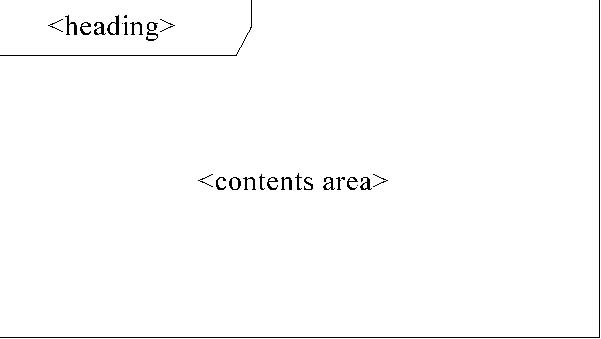Figure A.1

The frame is a rectangle. The frame is primarily used in cases where the diagrammed element has graphical border elements, like ports for classes and components (in connection with composite structures), and entry/exit points on statemachines. In cases where not needed, the frame may be omitted and implied by the border of the diagram area provided by a tool. In case the frame is omitted, the heading is also omitted.

The diagram contents area contains the graphical symbols; the primary graphical symbols define the type of the diagram (e.g., a class diagram is a diagram where the primary symbols in the contents area are class symbols).

The heading is a string contained in a name tag (rectangle with cutoff corner) in the upper leftmost corner of the rectangle, with the following syntax:

[<kind>]<name>[<parameters>]

The heading of a diagram represents the kind, name, and parameters of the namespace enclosing or the model element owning elements that are represented by symbols in the contents area. Most elements of a diagram contents area represent model elements that are defined in the namespace or are owned by another model element.

As an example, Figure A.2 is a class diagram of a package P: classes C1 and C2 are defined in the namespace of the package P.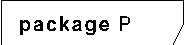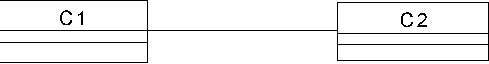Figure A.2 - Class diagram of package P

Figure A.3 illustrates that a package symbol for package P (in some containing package CP) may show the same contents as the class diagram for the package. i) is a diagram for package CP with graphical symbols representing the fact that the CP package contains a package P. ii) is a class diagram for this package P. Note that the package symbol in i) does not have to contain the class symbols and the association symbol; for more realistic models, the package symbols will typically just have the package names, while the class diagrams for the packages will have class symbols for the classes defined in the packages.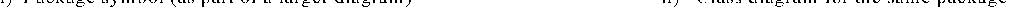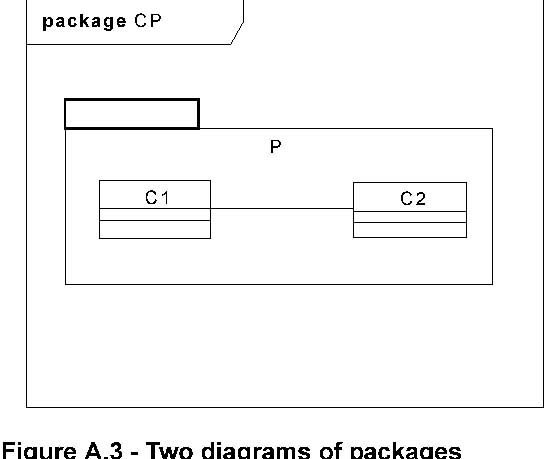Figure A.3 - Two diagrams of packages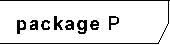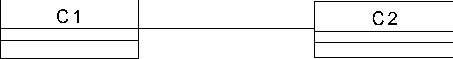In Figure A.4 i) is a class diagram for the package Cars, with a class symbol representing the fact that the Cars package contains a class Car. ii) is a composite structure diagram for this class Car. The class symbol in i) does not have to contain the structure of the class in a compartment; for more realistic models, the class symbols will typically just have the class names, while the composite structure diagrams for the classes will have symbols for the composite structures.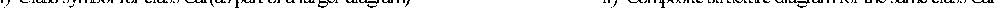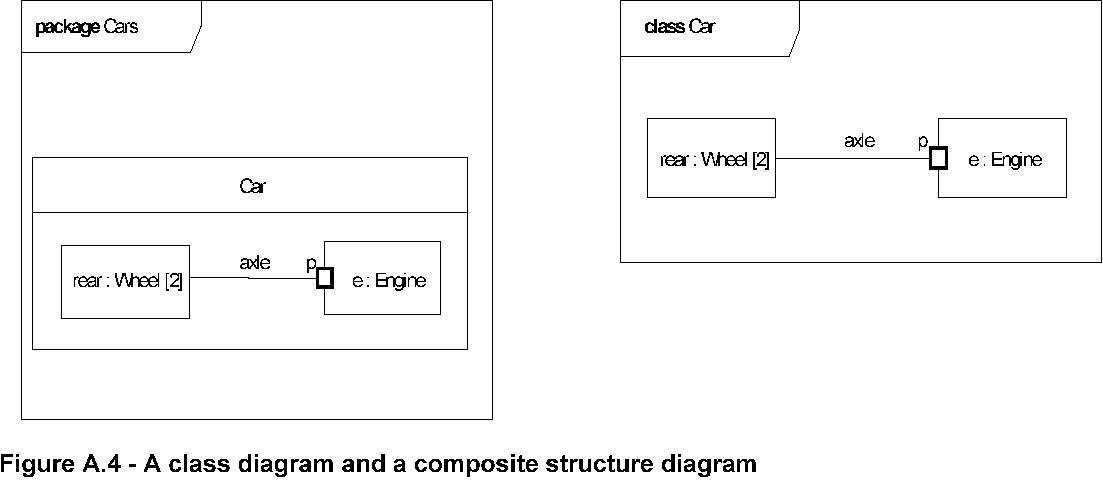Figure A.4 - A class diagram and a composite structure diagram

UML diagrams may have the following kinds of frame names as part of the heading:

activity

class

component

interaction

package

state machine

use case

In addition to the long form names for diagram heading types, the following abbreviated forms can also be used:

act (for activity frames)

cmp (for component frames)

sd (for interaction frames)

pkg (for package frames)

stm (for state machine frames)

uc (for use case frames)

As is shown in Figure A.5 , there are two major kinds of diagram types: structure diagrams and behavior diagrams.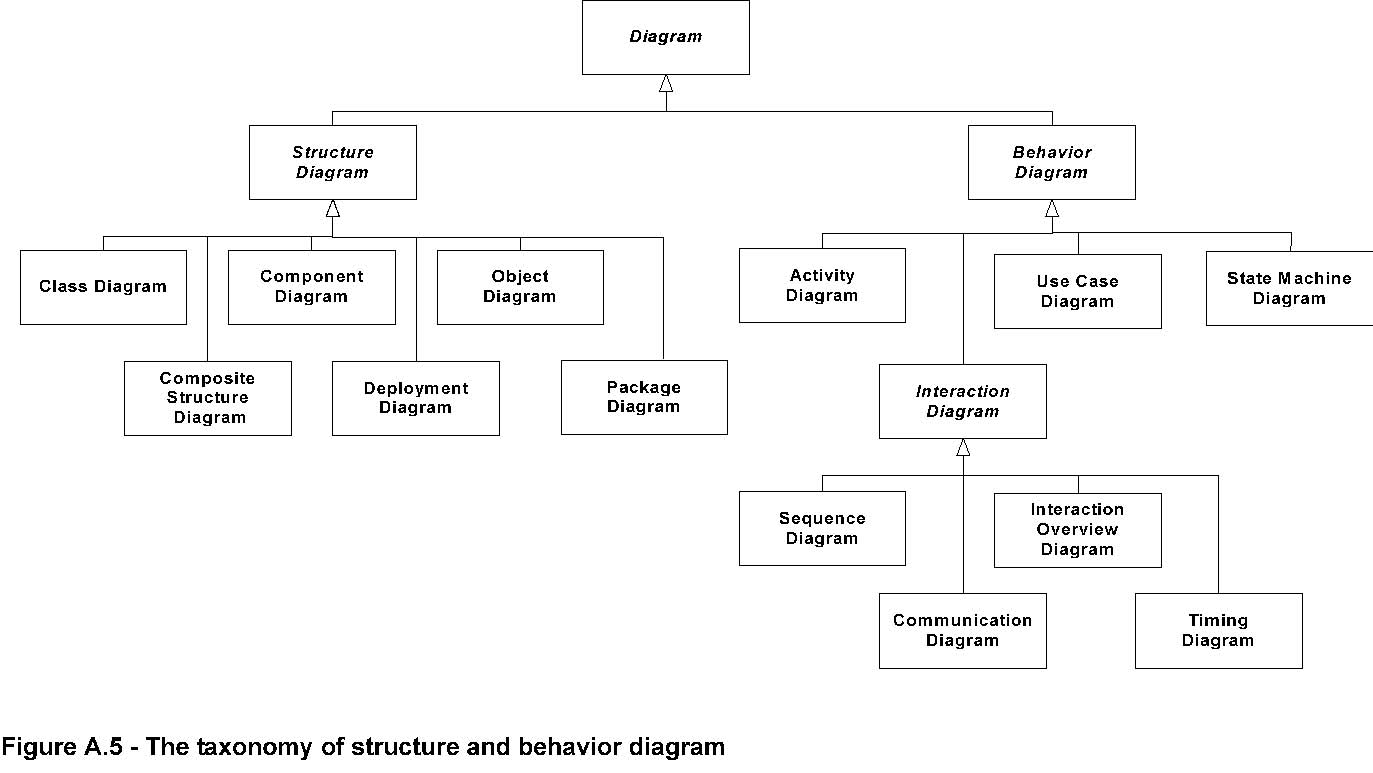Figure A.5 - The taxonomy of structure and behavior diagram

Structure diagrams show the static structure of the objects in a system. That is, they depict those elements in a specification that are irrespective of time. The elements in a structure diagram represent the meaningful concepts of an application, and may include abstract, real-world and implementation concepts. For example, a structure diagram for an airline reservation system might include classifiers that represent seat assignment algorithms, tickets, and a credit authorization service. Structure diagrams do not show the details of dynamic behavior, which are illustrated by behavioral diagrams. However, they may show relationships to the behaviors of the classifiers exhibited in the structure diagrams.

Behavior diagrams show the dynamic behavior of the objects in a system, including their methods, collaborations, activities, and state histories. The dynamic behavior of a system can be described as a series of changes to the system over time. Behavior diagrams can be further classified into several other kinds as illustrated in Figure A.5.

Please note that this taxonomy provides a logical organization for the various major kinds of diagrams. However, it does not preclude mixing different kinds of diagram types, as one might do when one combines structural and behavioral elements (e.g., showing a state machine nested inside an internal structure). Consequently, the boundaries between the various kinds of diagram types are not strictly enforced.

The constructs contained in each of the thirteen UML diagrams is described in the Superstructure chapters as indicated below.

Activity Diagram - Activities on page 307

Class Diagram - Classes on page 21

Communication Diagram - Interactions on page 477

Component Diagram - Components on page 147

Composite Structure Diagram - Composite Structures on page 167

Deployment diagram - Deployments on page 201

• Interaction Overview Diagram - Interactions on page 477

• Object Diagram - Classes on page 21

• Package Diagram -Classes on page 21

• State Machine Diagram - State Machines on page 545

• Sequence Diagram - Interactions on page 477

• Timing Diagram - Interactions on page 477

• Use Case Diagram - Use Cases on page 611